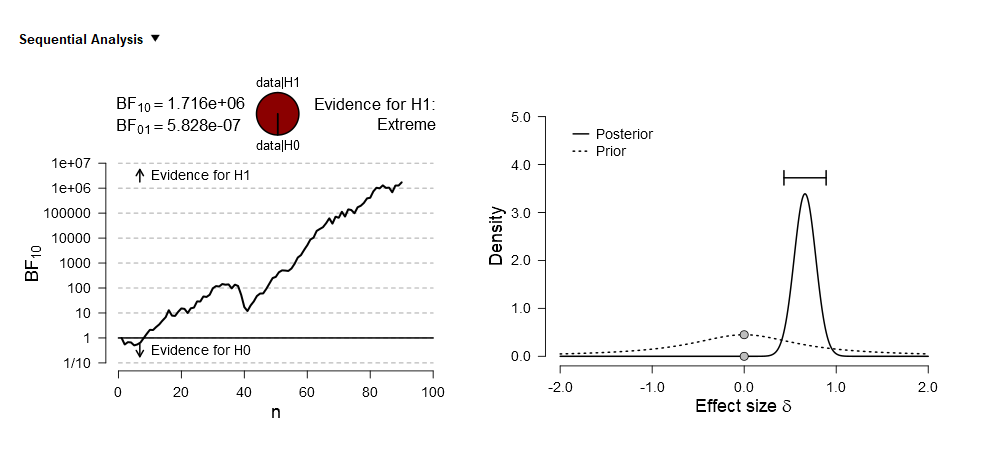#### Howdy, Stranger!

It looks like you're new here. If you want to get involved, click one of these buttons!

Supported by

# medium effect size but very large Bayes factor ?

I just received reviews of my first manuscript including Bayesian statistics (just for paired t-tests).
We got a (friendly) reviewer question - could someone help me phrase a correct answer or perhaps point me to a good reference?
"What I find difficult to understand is how you can have a medium effect size and a very strong Bayes Factor such as seen on page .., d = .60 & BF = 1,720,000."

• Hi HannaG,

What is relevant for the BF is the relative predictive performance of H0 vs H1. Unless d=0, the value of d actually tells you nothing at all about the BF; what you need is d and n. Specifically, when you have a low d but high n, you can still be pretty darn sure that H1 outpredicts H0. Conversely, a high d but very low n may only yield inconclusive evidence.

This is actually similar to NHST: you need both the sample effect size and the number of observations.
The equations for the BF have "n" in there; also, a prior-posterior plot will show exactly why you have this much evidence: the posterior must be relatively peaked and located away from zero; consequently, the posterior density at delta=0 is much reduced compared to the prior density at delta=0. I could go on, but basically the reviewer ignores sample size.

Note that this can be easily explored using the "Summary Stats" module in JASP (which requires that you enter the t-value and the sample size).

Cheers,
E.J.

• Thank you for taking the time to respond, I really appreciate it! The sample size was 'only' 90, so I am not sure if that counts as large and if this explanation holds. The plots also suggest that we got the high predictive performance of H1 already with relatively low n (see below).

Could it be about the consistency of the effect in the sample? Although I would expect that this would also be reflected in a large effect size (then again, 0.60 is not that small?). Hmm. We do get a very small p value < .000001 with a classic t-test.

So I am still a bit unsure what the explanation is and what an appropriate response to the reviewer would be. Would the following be accurate?
"BF summarizes the relative predictive performance of H0 vs H1. As in classic statistics, it is possible to obtain strong evidence also for a small effect size, if the sample size is large (see also the very small p-value of the t-test)
The value of BF depends on d, n, (and ...?). Our sample size was relatively high (N = 90) and the effect size of 0.60 (after correction for correlation between paired observations) was medium. This resulted in a large BF."• Yes, that's fine. But the effect is not small! So I would push back here. You have a medium effect size with a medium-to-large sample size. The result of that combination is displayed in the prior-posterior plot. Clearly an effect size of 0 is not supported by the data here (at all).

Thanked by 1HannaG
• edited October 2018

Thank you! Well then, I will try to convince our reviewer and myself with:
"BF summarizes the relative predictive performance of H0 vs H1. As in classic statistics, it is possible to obtain strong evidence in Bayesian statistics even for a small effect size, if the sample size is large enough (see also the very small p-value of the t-test). In this case, we have a medium effect size (d=0.60) with a medium-to-large sample size (N=90), and this resulted in a large BF. And the interpretation of this BF is that an effect size of 0 (H0) is clearly not supported by the data."

Thanked by 1EJ